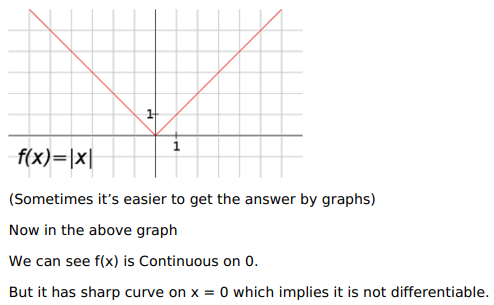# Solve this following

Question:

Mark $(\sqrt{)}$ against the correct answer in the following:

The function $f(\mathrm{x})=|\mathrm{x}| \forall \mathrm{x} \in \mathrm{R}$ is

A. continuous but not differentiable at $x=0$

B. differentiable but not continuous at $x=0$

C. neither continuous nor differentiable at $x=0$

D. none of these

Solution: